# Sphere VS

Find the surface and volume of a sphere that has a radius of 2 dm.

Correct result:

S =  50.2655 dm2
V =  33.5103 dm3

#### Solution: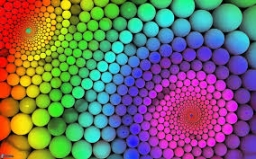We would be pleased if you find an error in the word problem, spelling mistakes, or inaccuracies and send it to us. Thank you!Tips to related online calculators
Tip: Our volume units converter will help you with the conversion of volume units.

#### You need to know the following knowledge to solve this word math problem:

We encourage you to watch this tutorial video on this math problem:

## Next similar math problems:

• Tulips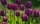Of the 120 tulips in the garden, 45 are red, 66 are yellow, the rest are brindle. What percentage of tulips in the garden are brindle?
• Seasonal saleThe bike became cheaper on the seasonal sale by 22%, which is 88 euros. At what price is it sold now?
• Pagans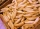Jano and Michael ate pagans. Jano ate three more than Michael. The product of their counts (numbers) is 180. How many pagans did each of them eat?
• 14 yearsKája is 14 years old. Mom 44. How many years will mom be 4 times older?
• Aircraft modelsHow many car models did the collector own after the collector's market? The collector of car and aircraft models owned 3 times more car models than aircraft. He sold 7 car models and 9 aircraft models on the stock exchange and bought 12 car models and 4 a
• Dance ensembles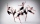4 dance ensembles were dancing at the festival. None had less than 10 and more than 20 members. All dancers from some of the two ensembles were represented in each dance. First, 31 participants were on the stage, then 32, 34, 35, 37, and 38. How many danc
• Operations with sets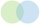The set B - A has twice as fewer elements than the set A - B and four times fewer elements than the set A ∩ B. How many times more elements does the set A have than the set B?
• An airplaneAn airplane flies 1440 km in 2 1/4 hours. What is its average speed in km per hour?
• Two shopsIn two different shops, the same skis had the same price. In the first, however, they first became more expensive by 20% and then cheaper by 5%. In the second, they were first cheaper by 5% and then more expensive by 30%, so after these adjustments in the
• One dice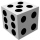Calculate the probability of a roll of one dice with the numbers 1, 2, 3, 4, 5, 6 on the walls. Write the results in a notebook in the shape of a fraction in the basic form: 2/3. a, The number 1 falls on the cube. b, The number 5 falls on the cube. c, An
• Subtracting sets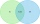For two sets K, L is true: K has 30 elements, L has 27 elements and the set L - K has 22 elements. How many elements does the set K - L have?
• Three diceThe player throwing the three dice asked G. Galilei the question: "Should I bet on the sum of 11 or the sum of 12?" What did Galilei answer him? Hint: write down all three triples of numbers that can be thrown and: have a total of 11 have a total of 12 an
• Prime divisorsFind 2/3 of the ratio of the sum and the product of all prime divisors of the number 120.
• GardensThe area of the square garden is 3/4 of the area of the triangular garden with sides of 80 m, 50 m, 50 m. How many meters of the fence do we need to fence a square garden?The three boys moved from start to finish on three different routes A, B, C, always at the same time. Adam drove road A 1500 m long on a scooter. Blake walked route B 600 m long on foot. Cyril got on a scooter on route C after a 90 m walk, then he left th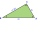In the acute triangle KLM, V is the intersection of its heights and X is the heel of height to the side KL. The axis of the angle XVL is parallel to the side LM and the angle MKL is 70°. What size are the KLM and KML angles?The gardening colony with dimensions of 180 m and 300 m is to be completely divided into equally large square areas with the largest possible area. Calculate how many such square areas can be obtained and determine the side length of the square.